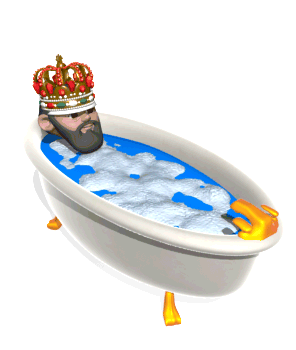# Buoyancy

As you can imagine, there is definitely more to whether an object floats or not than just average density. For example, why do some objects float higher in the water than others? And why is it easier to lift objects underwater than in the air? To answer these questions, you’ll need to understand the concept of buoyancy, a force which is exerted by a fluid on an object, opposing the object’s weight.

It is rumored that the Greek philosopher and scientist Archimedes, around 250 B.C., was asked by King Hiero II to help with a problem. King Hiero II had ordered a fancy golden crown from a goldsmith. However, the king was concerned that the goldsmith may have taken his money and mixed some silver in with the crown instead of crafting the crown out of pure gold. He asked Archimedes if there was a way to determine if the crown was pure gold.

Archimedes puzzled over the problem for some time, coming up with the solution while he was in the bath tub one evening. When Archimedes submerged himself in the tub, he noticed that the amount of water that spilled over the rim of the tub was equal to the volume of water he displaced.Using this method, he could place the crown in a bowl full of water. The amount of water that spilled over could be measured and used to tell the volume of the crown. By then dividing the mass of the crown by the volume, he could obtain the density of the crown, and compare the density to that of gold, determining if the crown was pure gold. According to legend, he was so excited he popped out of the tub and ran through the streets naked yelling “Eureka! Eureka!” (Greek for “I found it! I found it!”)

True story or not, this amusing tale illustrates Archimedes’ development of a key principle of buoyancy: the buoyant force (FB) on an object is equal to the density of the fluid, multiplied by the volume of the fluid displaced (which is also equal to the volume of the submerged portion of the object), multiplied by the gravitational field strength. This is known as Archimedes’ Principle.Archimedes’ Principle explains why boats made of steel can float. Although the steel of the boat itself is more dense than water, the average density of the entire boat (including the air in the interior of the boat) is less than that of water. Put another way, the boat floats because the weight of the volume of water displaced by the boat is greater than the weight of the boat itself.This principle also accounts for the ability of submarines to control their depth. Submarines use pumps to move water into and out of chambers in their interior, effectively controlling the average density of the submarine. If the submarine wants to rise, it pumps water out, reducing its average density. If it wants to submerge, it pumps water in, increasing its average density.

Question: What is the buoyant force on a 0.3 m3 box which is fully submerged in freshwater (density=1000 kg/m3)?

Answer:Question: A steel cable holds a 120-kg shark tank 3 meters below the surface of saltwater. If the volume of water displaced by the shark tank is 0.1 m3, what is the tension in the cable? Assume the density of saltwater is 1025 kg/m3.Answer: First, draw a free body diagram (FBD) of the situation, realizing that you have the force of gravity (mg) pulling down, the buoyant force upward, and the force of tension in the cable upward.

Because the shark tank is at equilibrium under the water, the net force on it must be zero, therefore the upward forces must balance the downward forces. You can write this using Newton’s 2nd Law in the y-direction as:Finally, you can use this equation to solve for the force of tension in the cable.Question: A rectangular boat made out of concrete with a mass of 3000 kg floats on a freshwater lake (ρ=1000 kg/m3). If the bottom area of the boat is 6 m2, how much of the boat is submerged?

Answer: Because the boat is floating on the lake, the magnitude of the buoyant force must be equal to the magnitude of the weight of the boat. (FB=mg).

Since the boat is rectangular, you can write its volume (V) as its bottom area (A=6 m2) multiplied by the depth submerged (d).